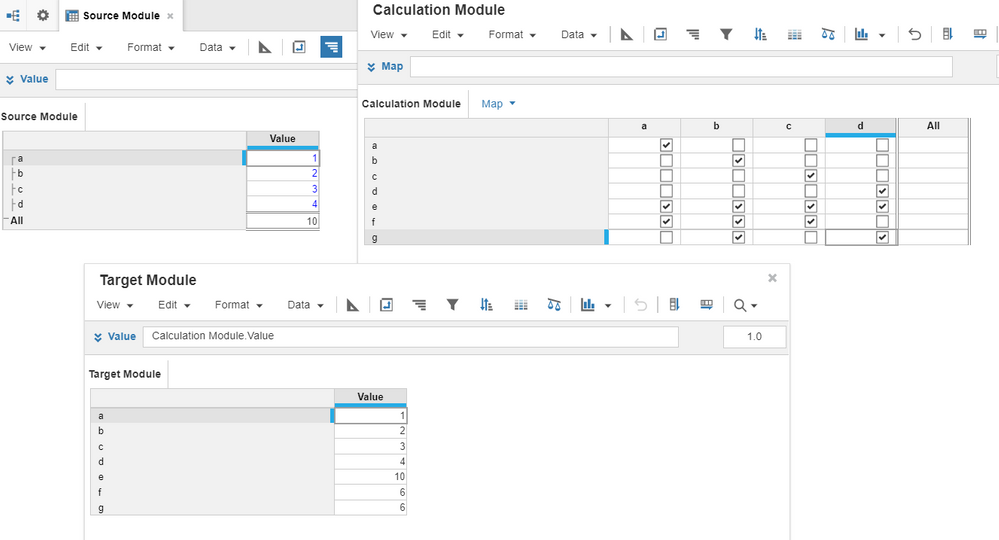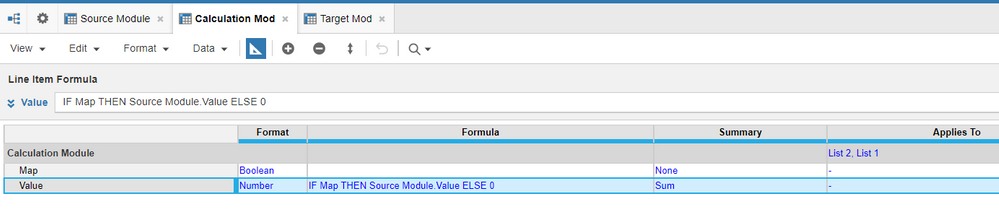# A list item value should be equal to the sum of values of other list items.Hi friends,

I am having a issue related to list items. As shown below, I have a list with items a, b, c, d. I have used this list as a dimension in a module which has value(number formatted) line item. I also have other list with items a, b, c, d, e, f, g.

Now, this list is used as a dimension in other module which also has value(number formatted) line item.

The issue is that I need to pull same values assigned to a, b, c, d list items but for remaining list items i.e. e, f, g.

I need the sum of previous list items i.e. e=a+ b+ c+ d i.e. '10' , f= a+ b+ c i.e. '6', g= b+ d i.e. '6'. Please help me with this issue.

Tagged:

•I have created a mapping table and two line items and using this I have got the result you expected. The reason I created this mapping table is because on the example you gave the formula for other list items like e,f,g and the formula looks random. So this way by creating mapping table and using the formulas in target module and calculation module I have put, you can easily get the expected result.Hope this helps.

Thanks

Tony

•Hi @Saisri8568

This is achievable, if it required for fewer list items. can you tell me how big the lists are, only a,b,c,d,e,f,g and other list only a,b,c,d?--> Finditem and lookup combination should help you.

If the lists are big and dynamic, apart from Sum, it is also possible.

Thanks,
Manjunath

•Yes, I used the same solution given by you but the original list(a, b, c, d,...) has nearly 15 list items and the second list(a, b, c, d, e, f, g....) has 30 list items. The formula became pretty lengthy, also I think it affects the performance.

•Hi

Can you share the formulas, we can think of refining it. and secondly if the lists are like 15 and 30. then you can use system module for achieving sum.

Thanks,
Manjunath

•Got this. But if in case we have multiple line items  in the module to pull data and more list items in the list, then sparsity will be increased right?. So, could we build some mapping module in the middle , using which we can sum source values?

•Got this. But if in case we have multiple line items in the module to pull data and more list items in the list, then sparsity will be increased right?. So, could we build some mapping module in the middle , using which we can sum source values?
•Yes, but as I see there is no consistent formula which we can put for all the list items in your problem. I think there is no other way. We would be having the sparsity.
•Hi

You dont have to worry about the sparsity unless it is hampering your performance. Solution by Tony will have sparsity but it is by far the best performance oriented solution.

if you want to reduce some sparsity, you may create subset to avoid one to one mapping between lists and get only sum values that are not one to one.(like initial 4 mappings in tony solution.)

Thanks
Manjunath